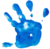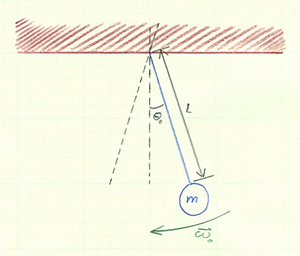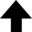Truth is ever to be found in simplicity, and not in the multiplicity and confusion of things.Isaac Newton

A common example of a mechanical system that exhibits harmonic motion is the simple pendulum. A simple pendulum is an idealized system consisting of a bob of mass $m$ attached to a piece of string of length $L$. The equation of motion of this system is

 $\displaystyle{ \dot{\theta}^2 =\frac{2 g}{L} \cos \theta + C \text{,} }$

where $C$ is a constant that is determined by the initial conditions of the pendulum. Two things to note about the differential equation above: 1) It is independent of mass $m$, and 2) it contains a useful parameter $g/L$ that the program will make use of as g_over_L. It is perhaps not surprising that the equation of motion is independent of mass since a simple laboratory experiment will reveal that altering the mass of the bob will have no effect on the period of the pendulum (more on the period in the Notes). Moreover, we can encode this fact into the algorithm by using Newton's second law in "reduced units" with a(i) = f_osc. This also has the advantage of reducing the number of operations the simulation needs to perform.

The default parameters of this program generate a stable numerical solution when simulating large amplitude oscillations.

### Reference• Boas, Mary. Mathematical Methods in the Physical Sciences. Wiley: John Wiley & Sons, 1983. 478.

## Oscillations of a Pendulum

This program solves the equation for the total energy of a simple pendulum to illustrate conservation of mechanical energy for large oscillations.
$\displaystyle{ \frac{1}{2} m L^2 \left( \frac{\mathrm{d} \theta}{\mathrm{d} t} \right)^2 = m g L (\cos \theta - \cos \theta_0) }$Initial angle $\theta_0$ of the mass: degrees1 ≤ $\theta_0$ ≤ 90° Length of the pendulum, $L$: m0.1 ≤ $L$ ≤ 5.0 m Initial angular velocity $\omega_0$ of the mass: deg/sec0 ≤ $\omega_0$ ≤ 23 deg/sec Time-step $\mathrm{d} t$ of the system: sec0.001 ≤ $\mathrm{d} t$ ≤ 0.100 sec Period of the pendulum:  sec
0%
Current data point:

Legend: Angle (deg); Angular velocity (deg/sec); Energy (J)